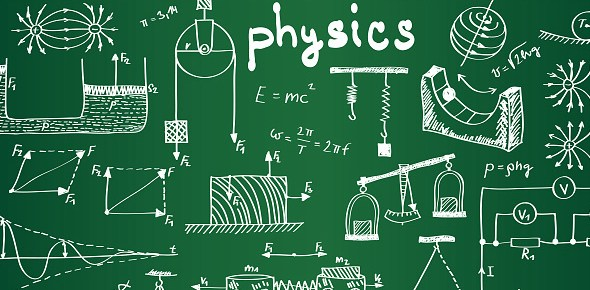# Physical Science Chapter 14

24 Questions | Attempts: 165
ShareSettingsPhysical science. Yes.

• 1.
The electron has no mass
• A.

True

• B.

False

• 2.
The red photons found in the emission spectrum of atomic hydrogen are less energetic than the purple photons
• A.

True

• B.

False

• 3.
The rare, backward reflection of alpha particles convinced Rutheford that the atom consisted of a dense, positively- charged nucleus.
• A.

True

• B.

False

• 4.
In gas discharge tube experiments, positive fragments from different types of atoms are similar to one another.
• A.

True

• B.

False

• 5.
To explain the continuous spectrum, Planck proposed that light could only be absorbed  or emitted in discrete amounts.
• A.

True

• B.

False

• 6.
Bohr failed to explain why an accelerated electron does not radiate energy.
• A.

True

• B.

False

• 7.
Rutherford fully expected alpha particles to bounce off of the gold foil.
• A.

True

• B.

False

• 8.
The nucleus of an atom occupies more than half of the atom's volume.
• A.

True

• B.

False

• 9.
The color of a hot object is related to its temperature.
• A.

True

• B.

False

• 10.
Dense, positively- charged center of the atom.
• 11.
Rutherford model
• 12.
Spectra in which the colors of light change abruptly and many colors are missing
• 13.
Thomson Model
• 14.
Positively charged particle emitted by some radioactive materials
• 15.
The conservation of ________ law explains why photon emission occurs when an electron drops from a high to a low energy state.
• 16.
Thomson's and Millikan's experiments provided strong evidence for the atom's _________ nature.
• 17.
Rutherford's orbits were _________________: Bohr's orbits were __________.
• 18.
An electron with a large value of n in the Bohr model is ______________ from the nucleus and has ___________ of energy.
• 19.
The nature functions using discrete rather than continuous energy is an assumption of the _________ model.
• 20.
Thomson's gas discharge experiments provide direct experimental evidence for the
• A.

Exclusion principle

• B.

Nuclear model of atoms

• C.

Bohr model of atoms

• D.

Idea that all atoms are made of charged particles

• E.

Idea that electrons in atoms behave as waves

• 21.
In the Thomson (plum pudding) model of the atom, an atom is described as
• A.

A positive "pudding" with small lumps of negative charge distributed throughout

• B.

A negative "pudding" with small lumps of positive charge distributed throughout

• C.

A negative "pudding" with one large lump of positive charge in the middle

• D.

A neutral "pudding" with lumps of negative charge around the outside and lumps of positive charge in the middle

• 22.
In what model do electrons orbit the nucleus so that any orbit allowed by Newtonian physics can be occupied by an electron?
• A.

Quantum model

• B.

Thomson or "plum pudding" model

• C.

Rutherfod or "solar system" model

• D.

Bohr or "modified solar system" model

• E.

Molecular model

• 23.
White light passes through a gas that absorbs some of the light. The spectrum of light that passes through the gas is then analyzed. The spectrum could best be described as
• A.

Continuous

• B.

A discrete spectrum of colored lines

• C.

A mostly continuous spectrum that is missing some colors

• D.

A discrete spectrum of colored lines that corresponds to the absorbed light

• E.

A completely black spectrum

• 24.
Gases emit light when they are energized by an electrical discharge. The light results when an electron
• A.

"jumps" from one energy level up to a higher energy level

• B.

"jumps" from one energy level down to a lower energy level

• C.

Is completely removed from the atom

• D.

Is absorbed b the nucleus

## Related TopicsBack to top
×

Wait!
Here's an interesting quiz for you.